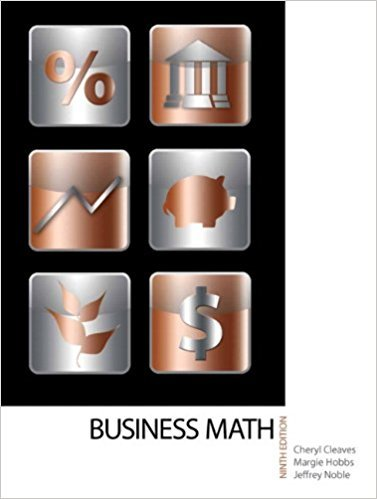×
×

# Solutions for Chapter 15-1: STOCKS## Full solutions for Business Math, | 9th Edition

ISBN: 9780135108178Solutions for Chapter 15-1: STOCKS

Solutions for Chapter 15-1
4 5 0 360 Reviews
27
1
##### ISBN: 9780135108178

Since 18 problems in chapter 15-1: STOCKS have been answered, more than 17969 students have viewed full step-by-step solutions from this chapter. Business Math, was written by and is associated to the ISBN: 9780135108178. Chapter 15-1: STOCKS includes 18 full step-by-step solutions. This expansive textbook survival guide covers the following chapters and their solutions. This textbook survival guide was created for the textbook: Business Math, , edition: 9.

Key Math Terms and definitions covered in this textbook
• Adjacency matrix of a graph.

Square matrix with aij = 1 when there is an edge from node i to node j; otherwise aij = O. A = AT when edges go both ways (undirected). Adjacency matrix of a graph. Square matrix with aij = 1 when there is an edge from node i to node j; otherwise aij = O. A = AT when edges go both ways (undirected).

• Back substitution.

Upper triangular systems are solved in reverse order Xn to Xl.

• Characteristic equation det(A - AI) = O.

The n roots are the eigenvalues of A.

• Cofactor Cij.

Remove row i and column j; multiply the determinant by (-I)i + j •

• Diagonal matrix D.

dij = 0 if i #- j. Block-diagonal: zero outside square blocks Du.

• Dimension of vector space

dim(V) = number of vectors in any basis for V.

• Factorization

A = L U. If elimination takes A to U without row exchanges, then the lower triangular L with multipliers eij (and eii = 1) brings U back to A.

• Fundamental Theorem.

The nullspace N (A) and row space C (AT) are orthogonal complements in Rn(perpendicular from Ax = 0 with dimensions rand n - r). Applied to AT, the column space C(A) is the orthogonal complement of N(AT) in Rm.

• Identity matrix I (or In).

Diagonal entries = 1, off-diagonal entries = 0.

• Independent vectors VI, .. " vk.

No combination cl VI + ... + qVk = zero vector unless all ci = O. If the v's are the columns of A, the only solution to Ax = 0 is x = o.

• Least squares solution X.

The vector x that minimizes the error lie 112 solves AT Ax = ATb. Then e = b - Ax is orthogonal to all columns of A.

• Markov matrix M.

All mij > 0 and each column sum is 1. Largest eigenvalue A = 1. If mij > 0, the columns of Mk approach the steady state eigenvector M s = s > O.

• Positive definite matrix A.

Symmetric matrix with positive eigenvalues and positive pivots. Definition: x T Ax > 0 unless x = O. Then A = LDLT with diag(D» O.

• Pseudoinverse A+ (Moore-Penrose inverse).

The n by m matrix that "inverts" A from column space back to row space, with N(A+) = N(AT). A+ A and AA+ are the projection matrices onto the row space and column space. Rank(A +) = rank(A).

• Skew-symmetric matrix K.

The transpose is -K, since Kij = -Kji. Eigenvalues are pure imaginary, eigenvectors are orthogonal, eKt is an orthogonal matrix.

• Spanning set.

Combinations of VI, ... ,Vm fill the space. The columns of A span C (A)!

• Spectrum of A = the set of eigenvalues {A I, ... , An}.

Spectral radius = max of IAi I.

• Standard basis for Rn.

Columns of n by n identity matrix (written i ,j ,k in R3).

• Subspace S of V.

Any vector space inside V, including V and Z = {zero vector only}.

• Vandermonde matrix V.

V c = b gives coefficients of p(x) = Co + ... + Cn_IXn- 1 with P(Xi) = bi. Vij = (Xi)j-I and det V = product of (Xk - Xi) for k > i.

×